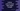# Python 3 program to print inverted right angled triangle## Introduction :

In this python programming tutorial, we will learn how to print an inverted right-angled triangle programmatically. A right-angled triangle has one 90 degrees angle or right angle. For an inverted right-angled triangle, this right angle will be at the top left corner. The other angles will be 45 degrees each in our example.

We will learn how to print the right-angled triangle using numbers or using any other characters. The program will ask the user to enter the height of the triangle if we are printing it using numbers. It will then print each row using numbers.

Similarly, if we are printing the triangle using other characters, it will ask the user to enter both height and character to use for the triangle.

Let me show you both of these approaches one by one :

### Python 3 program to print inverted right-angled triangle using number :

``````#example 1
height = int(input("Enter the height of the triangle : "))

for i in range(1,height+1):
for j in range(1,height - i+2):
print(str(j)+" ", end='')
print()``````
``````#example 2
height = int(input("Enter the height of the triangle : "))
c = str(input("Enter the character you want to print the triangle : "))

for i in range(0,height):
for j in range(0,height - i):
print(c+" ", end='')
print()``````

### How does it work?

In this example, we are printing an inverted right-angled triangle using only numbers. The numbers are printed serially like 1,2,3,4,5….. If the height is 5: for the first line, we are printing 5 numbers ‘1,2,3,4,5’, for the second line, we are printing 4 numbers ‘1,2,3,4’, for the third line, three numbers ‘1,2,3’ etc.

• First, we are taking the height of the triangle as an input from the user. The program can print a triangle of any height.
• We are running one for loop for height number of times. Inside this loop, we will print the characters for each row.
• For each iteration of the loop, we are running one more internal loop to print the numbers of the triangle. This is an inner for-loop. For each iteration of the outer loop, the inner loop will run.
• The inner loop runs from j = 1 to j = height – i +2 i.e. if height is 5, for first time, it will run in range j = 1 to j = 5 – 1 + 2 = 6 or for 5 times, for the second time, it will run from j = 1 to j = 5 or for 4 times etc.
• The inner loop prints out the numbers serially.

We can also print the same inverted triangle using any character. Let’s check :

### How does it work?

This example is the same as the above one. We are running two ‘for’ loops: the outer one will run for the same number of times as the height of the triangle, and the inner one will run based on the current height. Unlike the previous example, we are printing a character to create the triangle. The character is also given by the user. Here, we are using ‘*’ to create the triangle, but we can use ‘\$’,’&’,’#’ etc.

### Conclusion :

In this tutorial, we have learned how to print one inverted right-angled triangle in python using numbers or any other characters. This tutorial is a good example of using nested for loops in python. You can modify the program to print one non-inverted right-angle triangle. You can also try to print any other shapes using the same approach.

Try to run the example program shown above and drop one comment below if you have any queries.